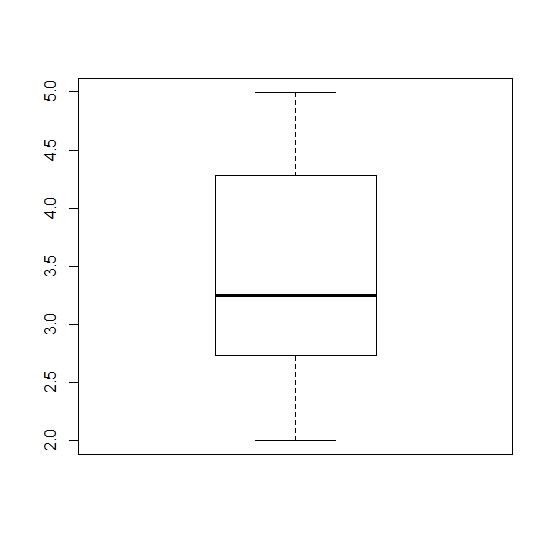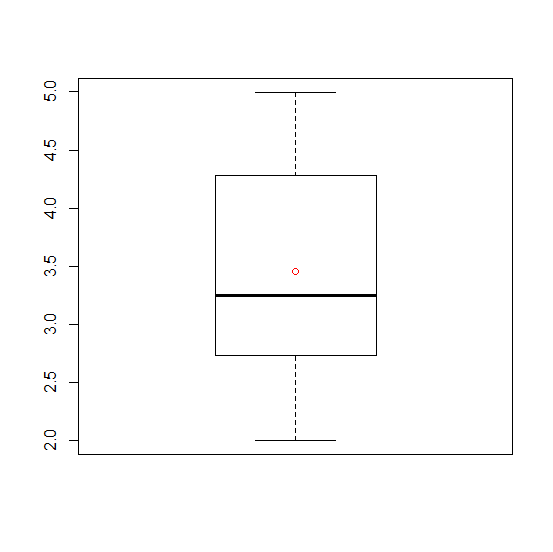# How to display mean inside boxplot created by using boxplot function in R?

A boxplot shows the median as a measure of center along with other values but we might want to compare the means as well. Therefore, showing mean with a point is likely to be preferred if we want to compare many boxplots. This can be done by using points(mean(“Vector_name”)), if we are plotting the columns of an R data frame then we will reference them instead of vector name.

## Example

Consider the below data and the boxplot −

Live Demo

x<-runif(100,2,5)
boxplot(x)

## OutputAdding mean point to the boxplot −

## Example

points(mean(x),col="red")


## Output